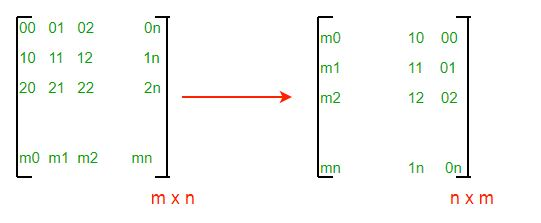# Turn an image by 90 degree

• Difficulty Level : Easy
• Last Updated : 30 Sep, 2019

Given an image, how will you turn it by 90 degrees? A vague question. Minimize the browser and try your solution before going further.

An image can be treated as 2D matrix which can be stored in a buffer. We are provided with matrix dimensions and it’s base address. How can we turn it?

Attention reader! Don’t stop learning now. Get hold of all the important DSA concepts with the DSA Self Paced Course at a student-friendly price and become industry ready.  To complete your preparation from learning a language to DS Algo and many more,  please refer Complete Interview Preparation Course.

In case you wish to attend live classes with experts, please refer DSA Live Classes for Working Professionals and Competitive Programming Live for Students.

For example see the below picture,

```* * * ^ * * *
* * * | * * *
* * * | * * *
* * * | * * *```

After rotating right, it appears (observe arrow direction)

```* * * *
* * * *
* * * *
-- - - >
* * * *
* * * *
* * * *```

The idea is simple. Transform each row of source matrix into required column of final image. We will use an auxiliary buffer to transform the image.

From the above picture, we can observe that

```first row of source ------> last column of destination
second row of source ------> last but-one column of destination
so ... on
last row of source ------> first column of destination```

In pictorial form, we can represent the above transformations of an (m x n) matrix into (n x m) matrix,Transformations

If you have not attempted, atleast try your pseudo code now.

It will be easy to write our pseudo code. In C/C++ we will usually traverse matrix on row major order. Each row is transformed into different column of final image. We need to construct columns of final image. See the following algorithm (transformation)

```for (r = 0; r < m; r++)
{
for (c = 0; c < n; c++)
{
// Hint: Map each source element indices into
// indices of destination matrix element.
dest_buffer [ c ] [ m - r - 1 ] = source_buffer [ r ] [ c ];
}
}```

Note that there are various ways to implement the algorithm based on traversal of matrix, row major or column major order. We have two matrices and two ways (row and column major) to traverse each matrix. Hence, there can atleast be 4 different ways of transformation of source matrix into final matrix.

## C++

 `// C++ program to turn an ``// image by 90 Degree ``#include ``using` `namespace` `std;``void` `displayMatrix(unsigned ``int` `const` `*p, ``                    ``unsigned ``int` `row, ``                   ``unsigned ``int` `col); ``                     ` `void` `rotate(unsigned ``int` `*pS, ``            ``unsigned ``int` `*pD, ``            ``unsigned ``int` `row, ``            ``unsigned ``int` `col); ``             ` `void` `displayMatrix(unsigned ``int` `const` `*p, ``                   ``unsigned ``int` `r, ``                   ``unsigned ``int` `c) ``{ ``    ``unsigned ``int` `row, col; ``    ``cout << ``"\n\n"``; `` ` `    ``for` `(row = 0; row < r; row++) ``    ``{ ``        ``for` `(col = 0; col < c; col++) ``            ``cout << * (p + row * c + col) << ``"\t"``; ``        ``cout << ``"\n"``; ``    ``} `` ` `    ``cout << ``"\n\n"``; ``} `` ` `void` `rotate(unsigned ``int` `*pS, ``            ``unsigned ``int` `*pD, ``            ``unsigned ``int` `row, ``            ``unsigned ``int` `col) ``{ ``    ``unsigned ``int` `r, c; ``    ``for` `(r = 0; r < row; r++) ``    ``{ ``        ``for` `(c = 0; c < col; c++) ``        ``{ ``            ``*(pD + c * row + (row - r - 1)) = ``                        ``*(pS + r * col + c); ``        ``} ``    ``} ``} `` ` `// Driver Code ``int` `main() ``{ ``     ` `    ``// declarations ``    ``unsigned ``int` `image[] = {{1, 2, 3, 4}, ``                               ``{5, 6, 7, 8}, ``                               ``{9, 10, 11, 12}}; ``    ``unsigned ``int` `*pSource; ``    ``unsigned ``int` `*pDestination; ``    ``unsigned ``int` `m, n; `` ` `    ``// setting initial values ``    ``// and memory allocation ``    ``m = 3, n = 4, pSource = (unsigned ``int` `*)image; ``    ``pDestination = (unsigned ``int` `*)``malloc``                   ``(``sizeof``(``int``) * m * n); `` ` `    ``// process each buffer ``    ``displayMatrix(pSource, m, n); `` ` `    ``rotate(pSource, pDestination, m, n); `` ` `    ``displayMatrix(pDestination, n, m); `` ` `    ``free``(pDestination); `` ` `    ``return` `0; ``} `` ` `// This code is contributed by rathbhupendra`

## C

 `// C program to turn an ``// image by 90 Degree``#include ``#include `` ` `void` `displayMatrix(unsigned ``int` `const` `*p, ``                   ``unsigned ``int` `row, ``                   ``unsigned ``int` `col);``                    ` `void` `rotate(unsigned ``int` `*pS, ``            ``unsigned ``int` `*pD, ``            ``unsigned ``int` `row, ``            ``unsigned ``int` `col);``             ` `void` `displayMatrix(unsigned ``int` `const` `*p, ``                   ``unsigned ``int` `r, ``                   ``unsigned ``int` `c) ``{``    ``unsigned ``int` `row, col;``    ``printf``(``"\n\n"``);`` ` `    ``for` `(row = 0; row < r; row++)``    ``{``        ``for` `(col = 0; col < c; col++)``            ``printf``(``"%d\t"``, * (p + row * c + col));``        ``printf``(``"\n"``);``    ``}`` ` `    ``printf``(``"\n\n"``);``}`` ` `void` `rotate(unsigned ``int` `*pS, ``            ``unsigned ``int` `*pD,``            ``unsigned ``int` `row, ``            ``unsigned ``int` `col)``{``    ``unsigned ``int` `r, c;``    ``for` `(r = 0; r < row; r++)``    ``{``        ``for` `(c = 0; c < col; c++)``        ``{``            ``*(pD + c * row + (row - r - 1)) = ``                            ``*(pS + r * col + c);``        ``}``    ``}``}`` ` `// Driver Code``int` `main()``{``     ` `    ``// declarations``    ``unsigned ``int` `image[] = {{1,2,3,4}, ``                               ``{5,6,7,8},``                               ``{9,10,11,12}};``    ``unsigned ``int` `*pSource;``    ``unsigned ``int` `*pDestination;``    ``unsigned ``int` `m, n;`` ` `    ``// setting initial values``    ``// and memory allocation``    ``m = 3, n = 4, pSource = (unsigned ``int` `*)image;``    ``pDestination = ``        ``(unsigned ``int` `*)``malloc` `        ``(``sizeof``(``int``) * m * n);`` ` `    ``// process each buffer``    ``displayMatrix(pSource, m, n);`` ` `    ``rotate(pSource, pDestination, m, n);`` ` `    ``displayMatrix(pDestination, n, m);`` ` `    ``free``(pDestination);`` ` `    ``getchar``();``    ``return` `0;``}`

Output :

```

1    2    3    4
5    6    7    8
9    10    11    12

9    5    1
10    6    2
11    7    3
12    8    4

```

Inplace rotate square matrix by 90 degrees